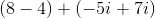Example Questions

Example Question #16 : Squaring / Square Roots / Radicals

Subtractfrom, given: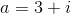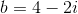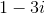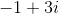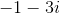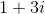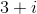Explanation:

A complex number is a combination of a real and imaginary number. To subtract complex numbers, subtract each element separately.

In equationis the real component andis the imaginary component (designated by). In equationis the real component andis the imaginary component. Solving for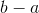,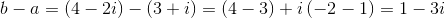Example Question #17 : Squaring / Square Roots / Radicals

Simplify the exponent,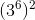.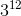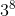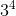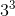Explanation:

When you have an exponent on the outside of parentheses while another is on the inside of the parentheses, such as in, multiply the exponents together to get the answer:.

This is different than when you have two numbers with the same base multiplied together, such as in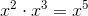. In that case, you add the exponents together.

Example Question #1 : Complex Numbers

Complex numbers take the form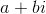, whereis the real term in the complex number and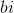is the nonreal (imaginary) term in the complex number.

Simplify: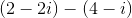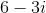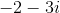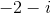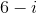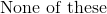Explanation:

Solving this equation is very similar to solving a linear binomial like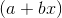. To solve, just combine like terms, being careful to watch for double negatives.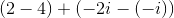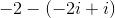Example Question #2 : Complex Numbers

Complex numbers take the form, whereis the real term in the complex number andis the nonreal (imaginary) term in the complex number.

Which of the following is incorrect?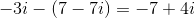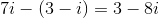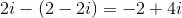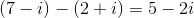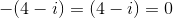Explanation:

A problem like this can be solved similarly to a linear binomial like/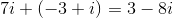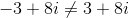Example Question #3 : Complex Numbers

Complex numbers take the form, whereis the real term in the complex number andis the nonreal (imaginary) term in the complex number.

Which of the following equations simplifies into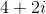?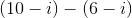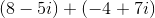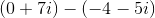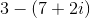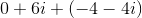Explanation:

This equation can be solved very similarly to a binomial like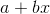.